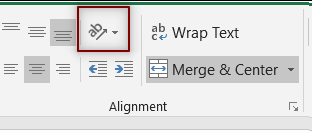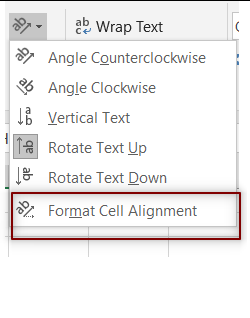PROVIDING ACCURATE, RELIABLE AND STRAIGHT TO THE POINT KNOWLEDGE ABOUT DIFFERENT IT TOOLS EXPLAINED WITH PRACTICAL EXAMPLES

# HOW TO ROTATE TEXT IN EXCEL

## INTRODUCTION

Whenever we prepare any report in excel, we have two constituents in any report.

The Text portion and the Numerical portion.

But just storing the text and numbers doesn’t make the super reports. Many times we need to automate the process in the reports to minimize the effort and improve the accuracy.

Many functions are provided by the Excel which work on Text and give us the useful output as well. But few problems are still left on which we need to apply some tricks with the available tools.

In this articles , we are going to learn many tricks and usage of function to perform different operations on the TEXT IN EXCEL.

## HOW TEXT IS HANDLED IN EXCEL?

TEXT is simply the group of characters and strings of characters which convey the information about the different data and numbers in Excel. Every character is connected with a code [ANSI].
Text comprises of the individual entity character which is the smallest bit which would be found in Excel.
We can perform the operations on the strings[Text] or the characters.
Characters are not limited to A to Z or a to z but many symbols are also included in this which we would see in the later part of the article.

TEXT IS AN INACTIVE NUMBER TYPE[FORMAT] IN EXCEL. ANYTHING STORED AS TEXT [NUMBER OR DATE] WON’T RESPOND TO ANY STANDARD FORMULAS OR FUNCTIONS BUT SPECIALLY DESIGNED TEXT FUNCTIONS. [EXCEPTIONS DO OCCUR IN CASE OF NUMBERS]
If we need to make anything inactive, such as Date to be non responding to the calculation, we put it as a text. Similarly if we want to avoid any calculations for a number it needs to be put as a text.

## WHY TO ROTATE TEXT IN EXCEL

Rotating the text means to give your text some inclination which can vary from 1 degree to 359 degree.

It means we can rotate the text to any angle . But the question arises, why would we rotate the text.

The Rotated text is the need of the report. We can rotate text from any of the following reason.

• Diagonal text needs less height and less width . So if we need to accommodate any lengthy text in the least space, the diagonal way is best and we need to rotate the text to get it diagonal aligned.
• Sometimes reports needs the vertically aligned Headings which can be easily provided using this option.
• Decorative purpose.

## ROTATE TEXT IN EXCEL

Let us learn to rotate text in Excel.

This can be the requirement of any report where we want to put the text as vertical or horizontal or at an angle.

It is quite simple as there is an option given for this in the Excel.

### STEPS TO ROTATE TEXT IN EXCEL:

• Select the text which we want to rotate.
• Go to HOME>ALIGNMENT>ORIENTATION• Click it and choose the angle for rotation . If we want to rotate to a specific angle, choose the option FORMAT CELL ALIGNMENT as shown in the picture below.• The animated picture below shows the complete process of rotating the text in excel.

• ## WHAT IS EXCEL, HOW EXCEL WORKS, WRITE FORMULA IN EXCEL, EXAMPLE OF USING FORMULA IN EXCEL, ANIMATED EXAMLPE OF USING FORMULA IN EXCEL

LEARN

• EXCEL
• JAVASCRIPT
• MORE TO COME…

Encourage us by following at …

If you have any queries or suggestions or any problem which you would like us to solve for you, kindly mail at

[email protected]